SLAS708A September   2010  – September 2019

PRODUCTION DATA.

1. Features
2. Applications
3. Description
4. Revision History
5. Device Comparison Table
6. Pin Configuration and Functions
7. Specifications
8. Detailed Description
1. 8.1 Overview
2. 8.2 Functional Block Diagram
3. 8.3 Feature Description
4. 8.4 Device Functional Modes
5. 8.5 Programming
9. Application and Implementation
1. 9.1 Application Information
10. 10Power Supply Recommendations
11. 11Layout
12. 12Device and Documentation Support
13. 13Mechanical, Packaging, and Orderable Information

• RTE|16
• RTE|16

### 7.11 Typical Characteristics: ADS7947 (12-Bit)

At TA = 25°C, DVDD = 1.8 V, VREF = 2.5 V, and fSAMPLE = 2 MSPS (unless otherwise noted)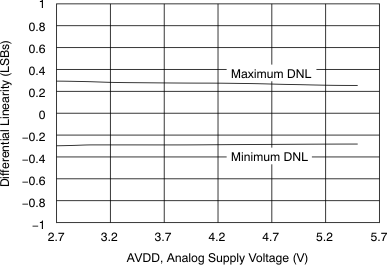Figure 7. Differential Linearity vs
Analog Supply Voltage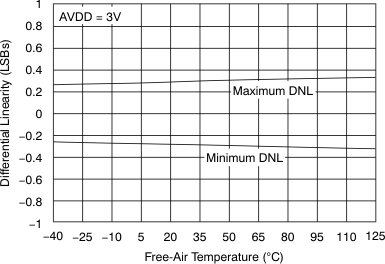Figure 9. Differential Linearity vs Temperature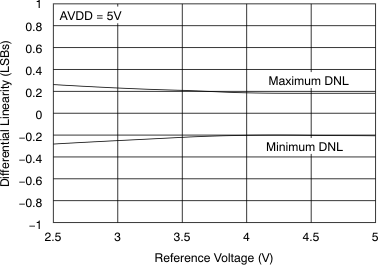Figure 11. Differential Linearity vs Reference Voltage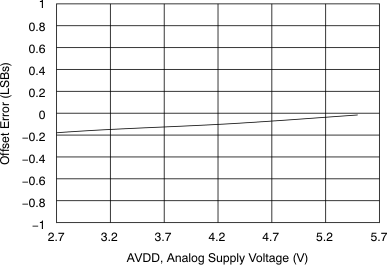Figure 13. Offset Error vs Analog Supply Voltage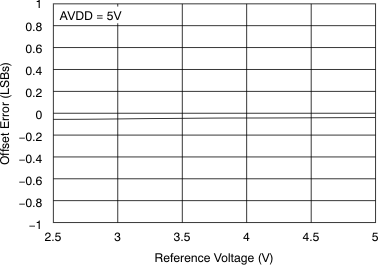Figure 15. Offset Error vs Reference Voltage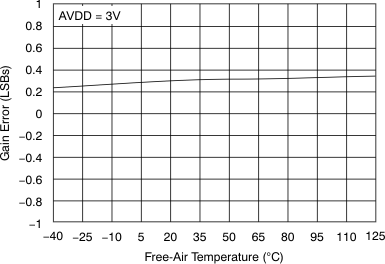Figure 17. Gain Error vs Temperature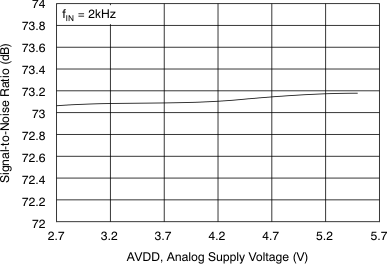Figure 19. SNR vs Analog Supply Voltage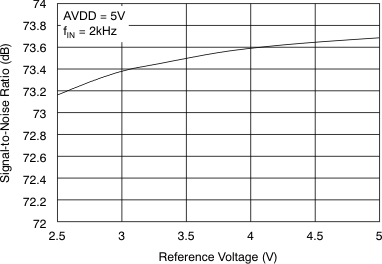Figure 21. SNR vs Reference Voltage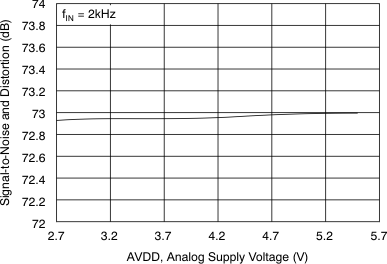Figure 23. SINAD vs Analog Supply Voltage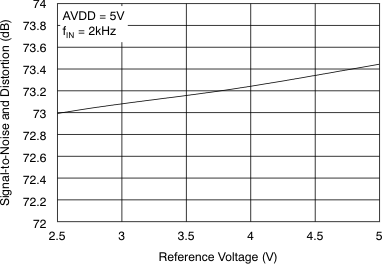Figure 25. SINAD vs Reference Voltage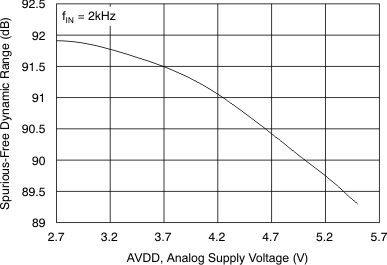Figure 27. SFDR vs AVDD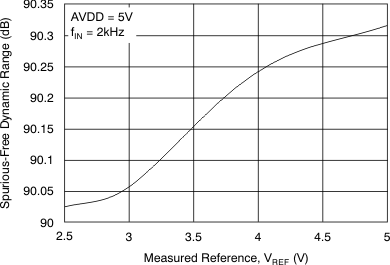Figure 29. SFDR vs Reference Voltage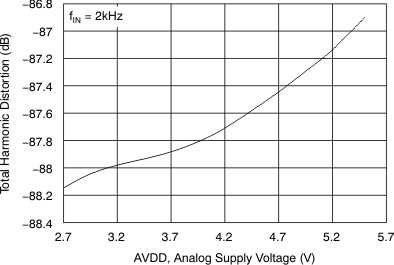Figure 31. SFDR vs AVDD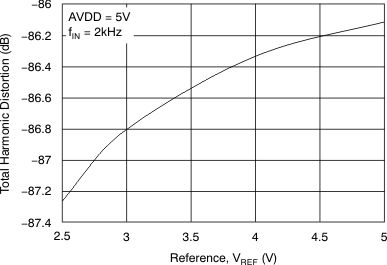Figure 33. THD vs Reference Voltage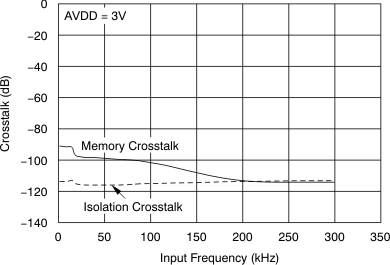Memory crosstalk is the effect of the last converted channel on the current converted channel data. Isolation crosstalk is the effect on the channel being converted that is coming from the signal on the channel that is off.
Figure 35. Crosstalk vs Input Frequency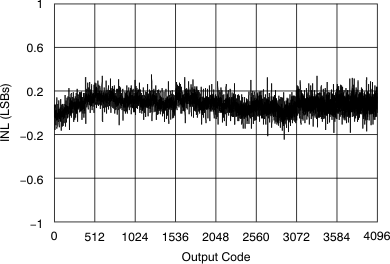Figure 37. Typical INL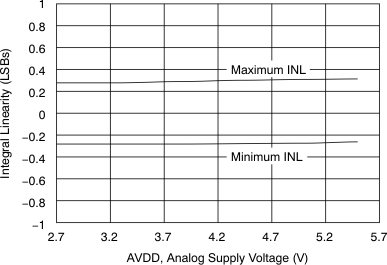Figure 8. Integral Linearity vs
Analog Supply Voltage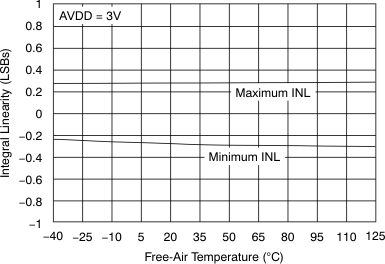Figure 10. Integral Linearity vs Temperature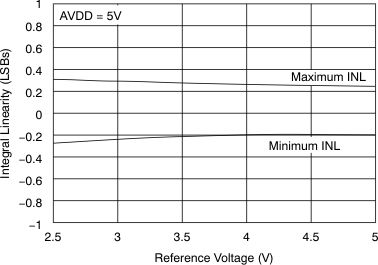Figure 12. Integral Linearity vs Reference Voltage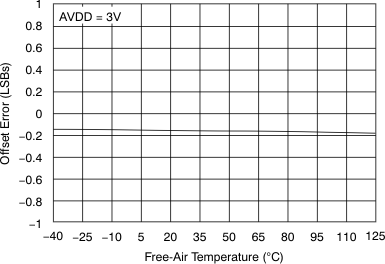Figure 14. Offset Error vs TemperatureFigure 16. Gain Error vs Analog Supply Voltage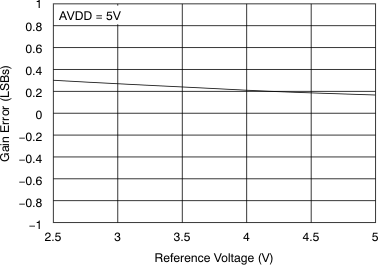Figure 18. Gain Error vs Reference Voltage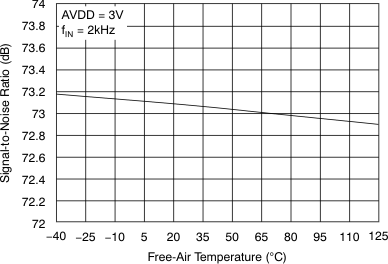Figure 20. SNR vs Temperature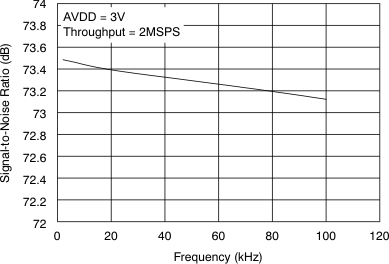Figure 22. SNR vs Input Frequency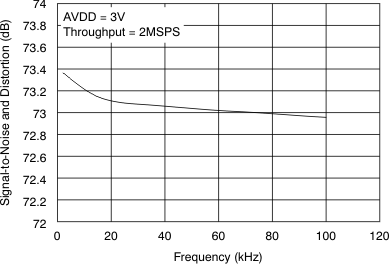Figure 26. SINAD vs Input Frequency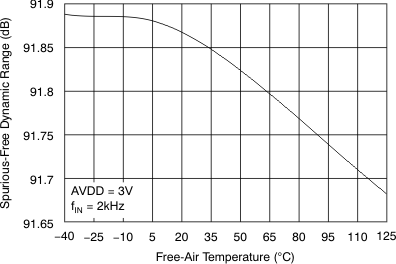Figure 28. SFDR vs Temperature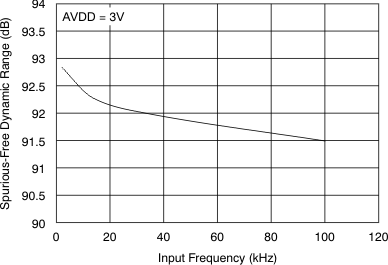Figure 30. SFDR vs Input Frequency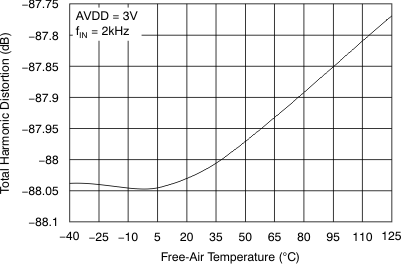Figure 32. SFDR vs Temperature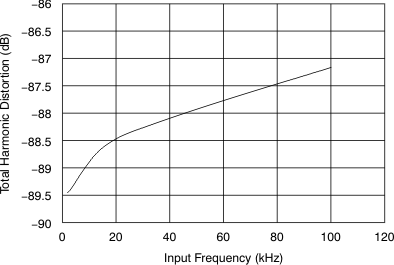Figure 34. THD vs Input Frequency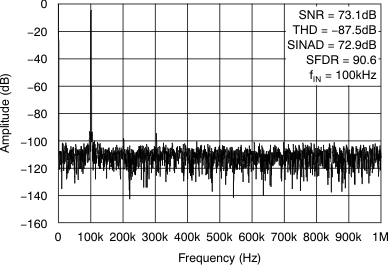Figure 36. Spectral Response
(8192-Point FFT)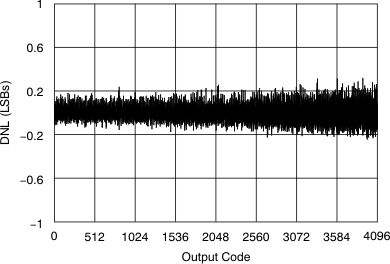Figure 38. Typical DNL